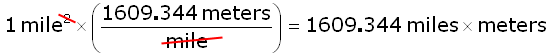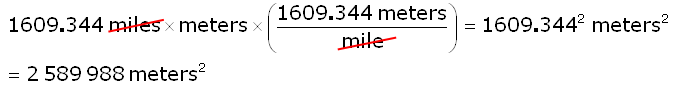SEARCH HOMEMath Central Quandaries & QueriesSubject: converting square miles to square meters Name: susan Who are you: Student how do you convert square miles into square metersHi Susan.

Each mile is 1609.344 meters. So this is the linear conversion factor. Let's do the arithmetic and show how we cancel the units. First we multiply by the conversion factor:Clearly we aren't done because the units are now miles times meters. So we do it again:This gives our answer.

Stephen La Rocque. >Math Central is supported by the University of Regina and The Pacific Institute for the Mathematical Sciences.